A Semi-Empirical Approach to Estimate Kinetic Parameters for the Batch and Continuous Settling of Flocculating Yeast Slurries | OMICS International
Journal of Bioprocessing & Biotechniques

Like us on:

All submissions of the EM system will be redirected to Online Manuscript Submission System. Authors are requested to submit articles directly to Online Manuscript Submission System of respective journal.

# A Semi-Empirical Approach to Estimate Kinetic Parameters for the Batch and Continuous Settling of Flocculating Yeast Slurries

Samuel Conceicao Oliveira1* and Reinaldo Giudici2

1Department of Bioprocesses and Biotechnology, Faculty of Pharmaceutical Sciences, UNESP, Rodovia Araraquara-Jaú km 1, Araraquara, Sao Paulo, Brazil

2Department of Chemical Engineering, Polytechnic School, University of Sao Paulo-USP, Av. Prof. Luciano Gualberto, Travessa 3, Sao Paulo, Brazil

*Corresponding Author:
Samuel Conceicao Oliveira
Department of Bioprocesses and Biotechnology
Faculty of Pharmaceutical Sciences, UNESP
Rodovia Araraquara- Jaú km 1, Araraquara, Sao Paulo, Brazil 14800-903
Tel: +551633014646
E-mail: [email protected]

Received Date: November 22, 2016; Accepted Date: November 28, 2016; Published Date: November 29, 2016

Citation: Oliveira SC, Giudici R (2016) A Semi-Empirical Approach to Estimate Kinetic Parameters for the Batch and Continuous Settling of Flocculating Yeast Slurries. J Bioprocess Biotech 6:290. doi: 10.4172/2155-9821.1000290

Copyright: © 2016 Oliveira SC, et al. This is an open-access article distributed under the terms of the Creative Commons Attribution License, which permits unrestricted use, distribution, and reproduction in any medium, provided the original author and source are credited.

Visit for more related articles at Journal of Bioprocessing & Biotechniques

#### Abstract

The knowledge of sedimentation kinetics is a necessary requisite for an adequate design of settlers aiming the separation and recovery of yeasts in alcoholic fermentation processes. In this study, batch and continuous settling tests were performed using slurries of flocculating yeasts obtained from the fermentation of sugarcane juice in a tower reactor with cell recycling. The batch tests were conducted in a 250 mL cylindrical glass vessel with initial concentrations of yeast between 90 and 100 g/L by measuring the variation over time of the height of the interface between the clarified liquid and the thickened slurry. These data were conveniently converted into cell concentration of the thickened sludge and a semi-empirical equation was developed to estimate the kinetic parameters of the settling. Data taken in a continuous settler in two continuous fermentation systems of different scales with cell recycle were also well fitted by the semi-empirical equation, but the adjusted parameters were different from those obtained for the batch tests.

#### Keywords

Semi-empirical modeling; Settling parameters; Flocculating yeast; Continuous ethanol fermentation; Cell recycle; Tower reactor

#### Introduction

Increased productivity is one of the most important objectives in continuous production of ethanol . It is generally accepted that a high concentration of yeast favors an increased ethanol yield of the fermentation process . Several techniques that promote the accumulation of biomass in fermenters have been used in continuous fermentation processes, including cell immobilization in polymeric matrices and cell recycle by centrifugation or filtration through membranes [2-4]. Good performances have been reported for processes using immobilized cells ; however, procedures and materials involved in this technique are inaccessible to a large number of small industrial units . Centrifugation and filtration equipments are extremely expensive and prone to mechanical problems; the use of these equipments is limited due to high investment and maintenance costs [2,5,6]. Since yeast separation by conventional methods is an expensive operation in alcoholic fermentation plants, alternative separation techniques using cheaper equipment such as hydrocyclones and settlers have been investigated aiming to reduce the costs involved in ethanol production [7-10].

Although solid particles can settle under the action of a centrifugal force, gravitational sedimentation is understood as the settling of particles within a fluid under the force of gravity. The factors that control the settling velocity are the solids concentration in the slurry, the densities of solid and liquid, the size and shape of the particles and the viscosity of the medium. However, the most important factors are the particle size and particle density.

The classical separation technique of gravitational sedimentation has not been used in fermentation processes due to the long time required for the settling of the cells. The main drawback of yeast separation is that these microorganisms are small in size and have densities only slightly higher than those of the reaction media in which they are suspended, resulting in very low settling velocities [7,10]. Thus, the major limitation for the use of gravitational sedimentation is the need for large-size tanks to allow a sufficient residence time for the cells to sediment .

According to Stokes law, large particles settle much faster than smaller ones, and overall sedimentation rates can thus be significantly affected by flocculation . Therefore, great practical advantages result from the increase of the particle size before sedimentation. In this scenario, the use of gravitational sedimentation processes in continuous fermentation with cell recycling becomes feasible when yeast strains that naturally form flocs are used [2,13]. These flocculating strains present much higher settling velocities than those of individual cells and hence can be efficiently separated even by small devices . Moreover, when scaling-up of the separation process is taken into account, sedimentation might be more feasible than other techniques due to its simplicity and operational stability as well as low cost and adequacy for any production scale .

Data of batch-settling tests can be used in the design of continuous thickeners. The main variables to be considered are the clarification efficiency and the height of the thickening zone, which depend on the cross-sectional area and the residence time in the tank (volume being the controlling factor), respectively.

Mathematical models for continuous thickeners are of great theoretical and practical interest in several applications such as bioprocesses with cell recycle. The rigorous mathematical modeling is based on the theory of mixtures of classical continuum mechanics. This theory describes a solid–liquid suspension as a mixture of two superimposed continuous media. Starting from the mass and linear momentum balances for each component, this theory yields three coupled partial differential equations that describe the sedimentation and consolidation processes of the suspension at the time and space . For the one-dimensional case, the mathematical model consists of an initial-boundary value problem for a second order partial differential equation. The nonlinear feature makes analytical solutions of these equations impossible, so usually numerical solutions are developed. The solutions may be discontinuous and difficulties arise when classical numerical methods are applied to obtain these solutions .

The excessive numerical effort involved in solving conservation equations (the main difficulty being the moving boundary of the suspension/sediment interface) makes the rigorous modeling more difficult to be used in the description of the dynamics of settling . For this reason, relatively simpler mathematical models, such as those based on semi-empirical approaches, have been preferred for practical applications. Semi-empirical modeling consists of building process models that are based on the experimental data interpretation and that are not fully formulated from the first principles (conservation laws, kinetic equations, thermodynamic equilibrium relationships, etc.).

In this study, batch- and continuous-settling tests were performed using slurries of flocculating yeasts obtained in a continuous fermentation process of ethanol production from sugarcane juice in a tower reactor with cell recycle. The objective was to develop and test a semi-empirical model for predicting the performance variables in continuous settlers used in continuous processes of alcoholic fermentation with cell recycle, specifically, the prediction of the cell concentration in the recycle stream to the fermenter (XS) as a function of the concentration of cells in the feeding of the settler (X) and the residence time of the slurry in the separation vessel (θS). The development of the semi-empirical model was based on the analysis of batch settling data. In addition, batch settling tests were also performed to investigate the differences between the settling kinetic parameters obtained in batch and those obtained in the continuous settling mode.

#### Materials and Methods

Batch settling tests

Six batch settling tests were conducted in a graduated cylinder using slurries of flocculating yeasts obtained from the fermentation of sugarcane juice in a continuous single-stage tower reactor with cell recycle. The tower fermentor (VF=0.22 L) was first inoculated with an initial yeast concentration of about 30 g/L. A period of 48 to 72 hours was required for the cell concentration to reach a value of around 100 g/L. At this stage of cell accumulation, the system was fed with solution of low sugar concentration (S0 ≅ 50 g/L) at a dilution rate (D) of 0.2 h-1. After reaching the steady state, the entire contents of the reactor (yeast suspension) were transferred to an Erlenmeyer flask and then homogenized for about 5 minutes by magnetic stirring. A sample was withdrawn for the determination of the initial concentration of the yeast slurry (C0), which was in the range between 90 and 100 g/L. The content of the Erlenmeyer flask was transferred to a 250 mL graduated cylinder and the time variation of the height of the interface between the clarified liquid and thickened slurry (sedimentation kinetics) was then monitored and registered.

Settling in the continuous system

Data for the continuous settling of flocculating yeast were measured in the continuous system described in our previous studies, in which continuous ethanol fermentation was performed in a tower reactor with recycle of flocculating yeast on two different scales. Details about the fermentation tests can be found in the mentioned references [17,18].

#### Results and Discussion

Determination of the settling parameters from batch tests

Figure 1 shows the variation with time of the height (Z) of the interface between the clarified liquid and thickened sludge measured during the batch settling tests. Two different periods can be observed in all tests. In the first period, the settling velocity, defined as dZ/dt, is practically constant, which is characterized by an almost linear decrease of the position of the interface with time. In the second period, the sedimentation velocity is variable and a nonlinear decay of Z(t) is observed (compression phase). The occurrence of these two distinct periods is typically observed in the literature for settling of concentrated slurries  (Figure 1).

Figure 1: Variation with time of the height of the interface between the clarified liquid and thickened sludge measured during the batch settling tests.

The linear period, in which the sedimentation rate is constant, can be modeled using the following equation: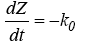(1)

where Z is the height of the interface between the clarified liquid and thickened slurry (cm), t is the time measured from the beginning of the test (min), and k0 is a zero-order kinetic constant (cm.min-1).

The nonlinear period, in which the sedimentation rate is variable, can be described by: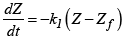(2)

where Zf is the final height of the interface between the clarified liquid and thickened slurry (cm) and k1 is a first-order kinetic constant (min-1).

Although these models are potentially able to describe the behaviour of the sedimentation curve separately in each period, the use of a single equation over the entire time of the test would be more suitable. Since that Equation 2 can also incorporate the settling behaviour at early times, their solution, subject to the initial condition Z=Z0 at t=0, is given by the following equation (for convenience, the subscript "1" in k1 has been dropped):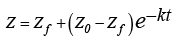(3)

Moreover, the height Z is related to the concentration of flocculating yeasts in the thickened sludge (C) by a simple mass balance of yeast, which results in: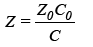(4)

Equation 4 is valid for all times of sedimentation, including the initial and final instants. Applying Equation 4 to the terms Z, Z0 and Zf in Equation 3 and simplifying the resulting equation gives: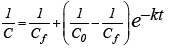(5)

where Cf is the maximum concentration of cells that can be achieved in the thickened slurry for t → ∞.

Figures 2 and 3 show the results for the fitting of Equation 5 to the data of each batch test separately and to the data of all tests simultaneously, respectively. The values of the adjusted parameters are indicated in these figures. Satisfactory agreement is observed, meaning that Equation 5 is able to describe correctly the trends of the experimental data.

Figure 2A: Fitting of the semi-empirical equation to the data of each batch test individually.

Figure 3A: Fitting of the semi-empirical equation to the data of all batch tests simultaneously.

For the purpose of using the adjusted parameters of the settling kinetics, it is recommended to employ those estimated using the data of all tests simultaneously, because the parameters thus obtained incorporate several sources of variation, such as differences in the viscosity of the medium, the density of yeast flocs, among other factors (Figures 2 and 3).

Determination of the settling parameters from data of the continuous fermentation process

Settling parameters for the continuous process were estimated by using the semi-empirical equation adapted for continuous sedimentation. By imposing phenomenological relationships of similarity between the main variables involved in each mode of sedimentation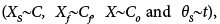Equation 6 can be established: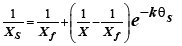(6)

where XS is the cell concentration in the stream recycled to the fermenter (stream leaving the bottom of the continuous settler), X is the cell concentration in the feed stream of the settler (equal to the cell concentration in the fermentor), θS is the residence time in the settler (= volume of the settler divided by the recycle flow rate) and Xf is the maximum cell concentration that could be achieved in the recycle stream (for very long residence times in the continuous settler).

In order to fit the semi-empirical equation (Equation 6) to the data acquired in the continuous process, the concentrations of yeast cells in the recycled stream XS were calculated from the mass balance of cells in the settler (Equation 7) with the data of X, Xe and αmeasured in the continuous fermentation process carried out on a larger scale, reported in our previous publication . The data are presented in Table 1.

D=F/VF(h-1) a (-) X (g/L) Xe (g/L) XS (g/L) 1/XS (L/g) qS (min)
0.11 10.3 101.4 0.1 111.2 0.00899 5.73
0.12 6.1 110.7 4.3 128.1 0.00781 8.86
0.16 5.5 94.1 5.6 110.2 0.00907 7.37
0.17 6.6 100.8 7.5 114.9 0.00870 5.78
0.18 10.4 93.1 10.0 101.1 0.00989 3.47
0.19 6.9 100.5 1.9 114.8 0.00871 4.95
0.20 6.4 94.2 8.7 107.6 0.00929 5.07
0.21 5.6 96.3 4.6 112.7 0.00887 5.52
0.22 18.0 98.7 4.3 103.9 0.00962 1.64
0.24 7.5 94.9 8.5 106.4 0.00940 3.60
0.25 7.5 95.8 3.3 108.1 0.00925 3.46
0.25 4.5 88.5 10.9 105.7 0.00946 5.77
0.27 5.4 90.4 3.9 106.4 0.00940 4.45
0.30 9.1 104.6 2.9 115.8 0.00864 2.38
0.33 9.7 99.6 2.7 109.6 0.00912 2.03

Table 1: Continuous sedimentation data (measured in a continuous fermentation process) for the fitting of the semi-empirical equation.

Assuming that the continuous settler schematically shown in Figure 4 is operated in steady-state conditions, the following mass balance equation for the cells can be written:

Figure 4: Schematic diagram of the continuous settler with indications of the flow rates and cell concentrations of the different streams.(7)

where α is the recycle ratio (=flow of recycle stream leaving the settler bottom divided by the outflow stream leaving the top of the settler), F is the volumetric flow rate of the clarified effluent stream leaving the top of the settler and Xe is the cell concentration in the clarified effluent (Table 1 and Figure 4).

Figure 5 shows the result of the fitting of the adapted semi-empirical equation (Equation 6) to the experimental data of continuous settling. Good agreement between the experimental and calculated data was obtained, even better than that observed for the fitting of the settling batch tests simultaneously (Figure 3B). However, the kinetic parameter k was significantly different for continuous settling and batch settling, as shown in Table 2 and Figure 5.

Figure 5: Parity plot for the fitting of the adapted semi-empirical equation to the experimental data of continuous settling obtained in the continuous fermentation process.

Parameters of the semi-empirical equation Batch settling
(Equation 5)
Continuous settling
(Equation 6)
Maximum reachable cell concentration in the thickened slurry: Cf or Xf(g/L) 187.5 138.8
k (min-1) 0.0129 0.107

Table 2: Estimated parameters of the semi-empirical equation for the batch and continuous settling.

The differences in the values of the parameters can be ascribed to the different time-scales of sedimentation involved in each case. While data were collected over a longer time span (0 to 4200 minutes) in the batch sedimentation tests, in the continuous runs the data covered values of residence times in a narrower range (2.03 to 8.83 minutes). The adjusting of the semi-empirical equation to continuous sedimentation data resulted in a smaller value for the maximum reachable concentration of cells in the thickened sludge Xf and a larger kinetic constant k in comparison to the corresponding parameters for the batch sedimentation tests.

The large difference (one order of magnitude) observed for the values of the kinetic parameter k for the batch tests and the continuous system deserves an explanation. According to the study of Tory and Shannon, the parameter k is not a true constant, but rather depends on the weight of solids per unit area, C0Z0, in such a way that k in Equations 3 and 5 can be written as k=b/(C0Z0), where b is the true kinetic constant of settling. If this is true, the slurry presents a settling rate that is a function only of the local solids concentration, thus obeying Kynch’s theory .

Moreover, even the value of the true constant b is expected to assume different values for the batch tests and the continuous system due to two reasons: (i) there are geometric differences between the two systems (volumes, height/diameter ratios) and (ii) there are important differences in the flux of the liquid. In the batch tests, there is a very slight ascending movement of the liquid as the solid particles settle, while in the continuous settler, at the region below the feeding position, the overall movement of the liquid is downwards (because most of the liquid exits the settler at its bottom with the recycle stream), thus imposing an additional downward drag force on the solids. Therefore, the value of b (and k) is expected to be greater for the continuous settler, as observed in the present study (Table 2).

Incidentally, the ratio between the two kinetic parameters, kcontinuous/ kbatch=8.3 was similar to the mean value of α (αm=8.0), thus suggesting directions for further investigations to clarify the relationship between these parameters. As a first approximation, the exponential term exp (-k*θS) was replaced by exp (-k’*α*θS) and the model was fitted again, being obtained a value of k’equal to 0.0189 min-1, of same magnitude order of kbatch, without impairing the predictive capability of the mathematical model, as evidenced in Figure 6.

Figure 6: Parity plot for the fitting of the adapted semi-empirical equation replacing the term exp(-k*θS) by exp(-k’*α*θS).

On the other hand, the observed values of the concentration factor w (defined as the ratio between the cell concentration in the bottom exit stream and in the settler feed, w=XS/X) were very low, close to 1.1 for the continuous settling systems on two different scales, as shown in Table 3. These low values of w indicate that the continuous settler was operated under conditions that did not concentrate the slurry to a great extent at the large recycle ratios (α) employed. Improvement of cell separation by optimization of the settler operating conditions will result in higher concentration factors, which is very desirable.

Process Scale a X Xe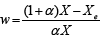small(a) 13.6 98.3 6.0 1.07
small 13.6 99.7 5.7 1.07
small 13.6 104.3 7.9 1.07
small 13.6 101.6 6.3 1.07
small 13.6 80.0 7.1 1.07
large(b) 10.1 101.4 0.1 1.10
large 6.1 110.7 4.3 1.16
large 5.5 94.1 5.6 1.17
large 6.6 100.8 7.5 1.14
large 10.4 93.1 10.0 1.09
large 6.9 100.5 1.9 1.14
large 6.4 94.2 8.7 1.14
large 5.6 96.3 4.6 1.17
large 18.0 98.7 4.3 1.05
large 7.5 94.9 8.5 1.12
large 7.5 95.8 3.3 1.13
large 4.5 88.5 10.9 1.19
large 5.4 90.4 3.9 1.18
large 9.1 104.6 2.9 1.11
large 9.7 99.6 2.7 1.10

Table 3: Values of the concentration factor obtained in two continuous fermentation processes on two different scales.

Limtong et al.  investigated the effect of the recycle ratio (α) on the concentration factor for flocculating yeast Saccharomyces cerevisiae TJ1 in a continuous alcoholic fermentation process similar in geometry and size to that studied here. They observed a nonlinear decay of this factor with increasing the recycle ratio, with an asymptotic value close to 1 being reached for α ≥ 4. Thus, since our runs of continuous fermentation were conducted with recycle ratios (α) greater than 4, as shown in Table 3, the concentration factor w is expected to be close to 1, as observed in Figure 7. Moreover, because w can be considered to be almost constant in this range of operating conditions, its best value could be estimated simultaneously with the fermentation kinetic parameters in the step of model fitting as done by de Oliveira et al.  when modeled a continuous alcoholic fermentation process in a twostage tower reactor cascade with flocculating yeast recycle (Figure 7 and Table 3).

Figure 7: Behavior of concentration factor (w) as a function of recycle ratio (α) in a continuous ethanol fermentation process with recycling of flocculating yeast.

#### Conclusion

From data collected in batch settling tests and an appropriate semi-empirical equation like the one developed in the present study, it was possible to determine kinetic parameters for the sedimentation of flocculating yeast slurries aiming the design of settlers used in continuous ethanol fermentation processes with cell recycle.

The semi-empirical equation developed for batch sedimentation was conveniently adapted and applied for continuous sedimentation by the establishment of phenomenological similarity relationships between the corresponding variables of the two sedimentation systems.

Parameters obtained from batch-wise tests were significantly different (mainly the parameter k) from those obtained in a continuous mode. Differences in residence times, geometry and liquid fluxes may further explain the observed discrepancies between the parameter values.

#### References

Select your language of interest to view the total content in your interested language

### Article Usage

• Total views: 1588
• [From(publication date):
November-2016 - Jul 23, 2019]
• Breakdown by view type
• HTML page views : 1459Can't read the image? click here to refresh